## Tables index

#### José Luís Pereira Rebelo Fernandes

International Journal of Physics. 2021, 9(1), 36-41 doi:10.12691/ijp-9-1-4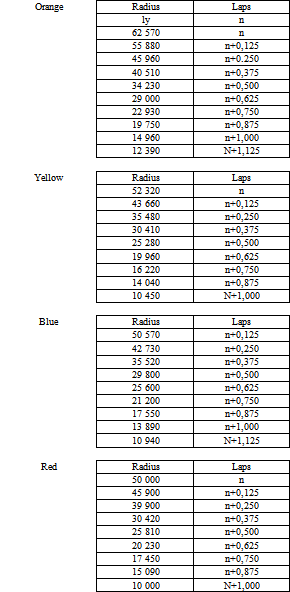• Table 1. Radius and nº of laps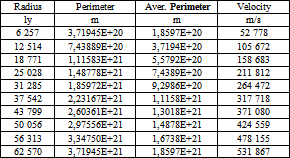• Table 2. Radius, Average Perimeter and Rotation Velocity (E->10^)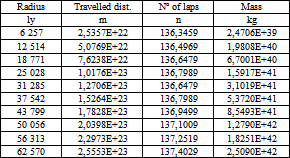• Table 3. Radius, Travelled distance, nº of laps and Mass (E->10^)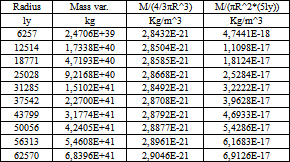• Table 4. Radius, Mass variation, Volume density, Density Accretion Disc with 5 ly thickness (E->10^)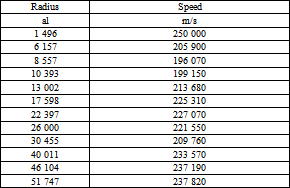• Table 5. Radius and Speeds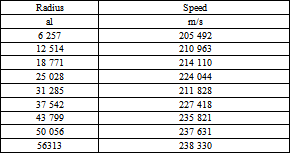• Table 6. - Radius and Speeds equivalents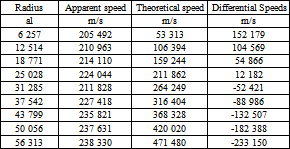• Table 7. Radius, Apparent speed. Theoretical speed and Differential Speeds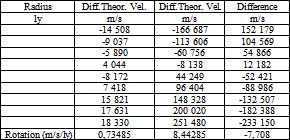• Table 8. Differential of speeds and respective rotation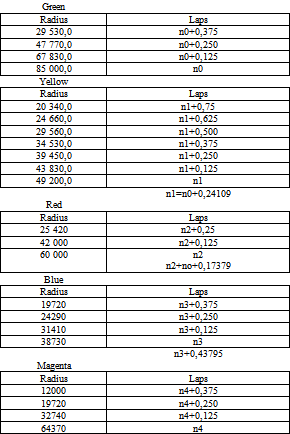• Table 9. Radius and nº of laps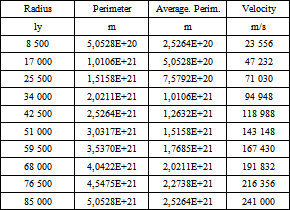• Table 10. Radius, Average Perimeter and Rotation Velocity (E->10^)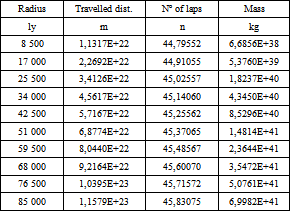• Table 11. Radius, Travelled distance, nº of laps and Mass (E->10^)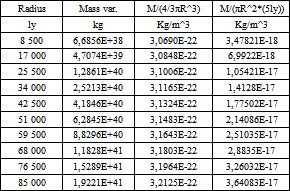• Table 12. Radius, Mass variation, Volume density, Density Accretion Disc with 5 ly thickness (E->10^)